This section is Section 2 of 3.

Speed as well as accuracy is important in this section. Work quickly, or you might not finish the paper. There are no  penalties for incorrect responses, only marks for correct answers, so you should attempt all 27 questions. Each question is worth one mark.

You must complete the answers within the time limit. Calculators are NOT permitted.

Good Luck!

Note – if press “End Exam” you can access full worked solutions for all past paper questions

Which row of the table correctly describes what happens when body temperature rises in a human?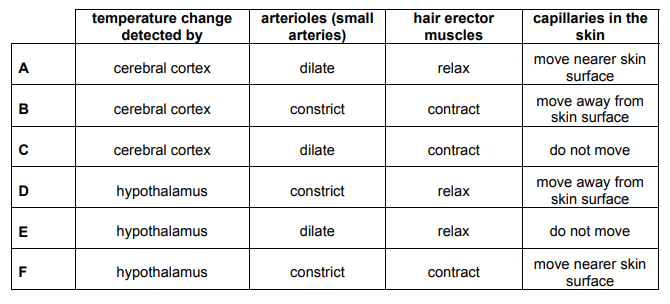1.
3
0

## Explanation

Temperature change is detected by the thermoregulatory centre in the hypothalamus. The thermoregulatory centre activates a response, in which: the arterioles dilate, hair erector muscles relax – be careful, the capillaries themselves do not move. However, dilation of the arterioles supplying blood to the skin capillaries, causes more warm blood to flow near the surface of the skin, hence heat is lost by radiation.

Post Comment

A compound of iodine and oxygen contains 63.5g of iodine and 20.0g of oxygen.

(Ar : I = 127; O = 16)

2. Which of the following would be its formula?
0
0

## Explanation

First, we need to calculate the moles of each element. Then, we need to compare their mole ratios to find the Relative Molecular

Formula (RFM).

n(I) = 63.5/127 = 0.5 ; n(O) = 20/16 = 1.25

0.5 : 1.25 1/2 : 5/4 (multiply by 4 on both sides) 2:5 ratio

Hence, 2I and 5O. Hence, the formula is: I₂O₅

Post Comment

In a laboratory experiment, protactinium-234 undergoes radioactive decay by β-emission into uranium-234.

The table below describes how the mass of uranium-234 present in the sample varies with time from the start of the experiment: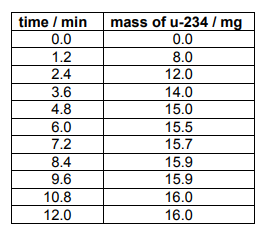3. Using the information in the table, approximately what is the half-life of protactinium-234?
0
0

## Explanation

The mass of uranium gained, corresponds to the mass of protactinium lost. Uranium’s final mass is 16mg, and half of this mass is 8mg. At 8mg, the time is 1.2 minutes, corresponding to the half life.

Post Comment

I have two containers with different capacities. Initially, the larger one is full of water and the smaller one is empty. I pour water from the larger container into the smaller container until they contain the same volume of water. The volume of water in the large container is now p times its capacity and the volume of water in the small container is q times its capacity.

4. Which one of the following statements about p and q must be true?
0
0

## Explanation

The big container is full to begin with. That counts as a ‘1’ unit of water. When the water is poured, you stop pouring until both containers have the same amount of water – the 1 unit is halved (0.5 + 0.5 = 1) to get 0.5 in each container. The big container has a capacity of 1 but only has 0.5 units of water in it, which is half of its capacity. Hence, p = 0.5. The smaller container now contains 0.5 units. Because it’s smaller than the big container, it must be more than half full. If the volume of water was half the capacity of the small container, you would times by 0.5. However, we know that the volume of water is more than half capacity. Therefore, we need to times capacity by more than 0.5 to find volume of water. Therefore, q must be bigger than 0.5. i.e q>0.5 Overall we now know that p=0.5 and q>0.5. p and q do not stand for the volume of water – they stand for what you times capacity is to find the volume of water. To find capacity of water in the big container:

Volume = capacity x p

Volume = 1 x 0.5

Volume = 0.5 .

Hence, p=0.5 and q>0.5.

Post Comment

The following statements are about hormone levels and their effect:

1 Increasing levels of insulin cause an increase in blood glucose levels.

2 Increasing levels of oestrogen increases the thickness of the inner lining of the uterus.

3 Increasing levels of adrenaline increases the heart rate.

5. Which of the statements are true?
0
0

## Explanation

Insulin is released in response to increased blood glucose concentration and acts to decrease blood glucose levels so statement 1 is false.

Statements 2 and 3 however are true.

Post Comment

Carbon, in the form of coke, is used to reduce iron oxide in a blast furnace. The three stages are shown below:

1 C + O₂ → CO₂

2 CO₂ + C → 2CO

3 3CO + Fe₂O₃ → 2Fe + 3CO₂

(Ar : C = 12; O = 16)

6. If 12g of carbon is used in stage 2 and all the carbon monoxide produced is used in stage 3, what mass of carbon dioxide is produced in stage 3?
0
0

## Explanation

2nd equation : CO2 + C 2CO

3rd equation : 3CO + Fe₂O₃ 2Fe + 3CO₂

Multiply the 3rd equation by 3 to give:

2CO + 2/3 Fe₂O₃ 4/3Fe + 2CO₂

12 C g represents 1 mole of C

The molar mass of CO2 is : Mr(CO₂) = 12 + 16(2) = 44

CO₂:2CO 1:2 mole ratio

2CO : 2CO₂ 1:1 mole ratio

1 mole of C gives 2 moles of CO₂

Hence, 2 x 44 = 88 g of CO₂

Post Comment

The depth of water in a particular tidal harbour varies with time as shown in the graph: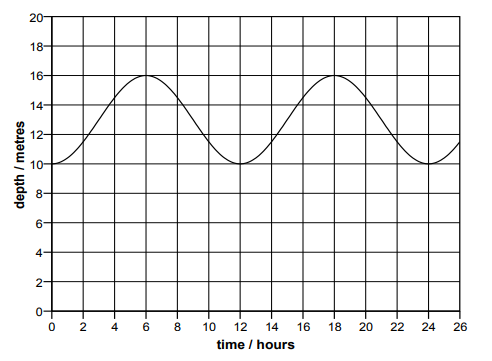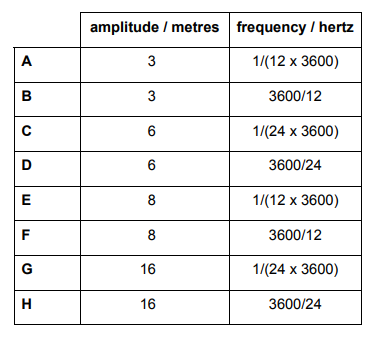7. If the variation in depth caused by the effect of the tide is considered as a wave, what are the amplitude and frequency of this wave?
0
0

## Explanation

The amplitude is 3, as the total height from maximum to minimum is 16-10 = 6, hence 6/2=3. This is as, amplitude is measured from the equilibrium position. The time period is the length of time from one minimum to another, or one maximum to another. Hence, this time: 12 hours. This is expressed in seconds = 12 x 3600s. The Frequency = 1/Time Period = 1/(12 x 3600)

Post Comment

A children’s game is played on a square grid starting in the centre. Players spin two spinners to decide how to move their counters. The first spinner decides the direction (Left, Right, Up or Down) and the second spinner decides the distance (1, 2, 3 or 4 squares).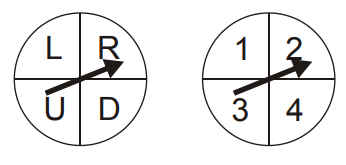8. What are the chances that, after two moves, a player is exactly back where they started?
0
0

## Explanation

There are 4 different possible outcomes per spinner, so there are 4×4=16 possible outcomes altogether. For a player to be moved back to where he/she started two moves ago, the second move must be exactly the reverse of the previous move. The chance of this is therefore 1/16.

Post Comment

Which one of the following correctly completes the statement:

During the process of evolution, natural selection will favour individuals with…

9.
0
0

## Explanation

During the process of evolution, natural selection will favour individuals with an advantageous allele. This is as, those with advantageous alleles outcompete individuals without advantages alleles, as they are more likely to survive, hence are more likely to breed successfully. Due to reproductive success, they are more likely to pass on their alleles to the next generation.

Post Comment

1 CH₄

2 CO

3 CO₂

4 H₂O

5 He

6 NH₃

10. Which two of the following are produced by the complete combustion of fuels?
0
0

## Explanation

The only products of hydrocarbon fuel complete combustion are: CO₂ and H₂O.

Post Comment

When radioactive isotopes decay, they sometimes have to go through a succession of disintegrations to reach a stable isotope. These are called decay chains, and involve the successive emission of numerous α and/or β particles.

One such isotope is radon-219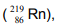which goes through a chain in which three α particles and two β particles are emitted before reaching a stable isotope.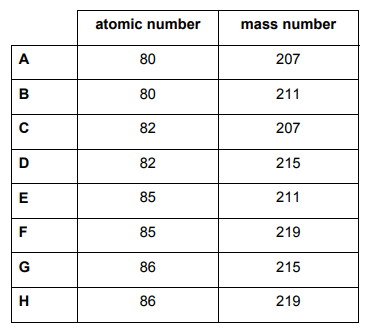11. What are the atomic and mass numbers of the resulting stable isotope?
0
0

## Explanation

Mass number: 219 – 3(4) – 2(0) =219 – 12 = 207

3 alpha particles have 4 nucleons each, hence 3 x 4 = 12, hence 12 nucleons lost.

Beta particles don’t cause a change in mass number, as a neutron turns into a proton, hence no change in nucleon number.

Atomic number: 86 – 3(2) + 2(1) = 82

3 alpha particles have 2 protons each, hence 3 x 2 = 6, hence 6 protons lost.

Beta particles change in proton number, as a neutron turns into a proton; as a proton is gained each time, twice, 2 protons are gained.

Post Comment

The mean time for running a race by a group of 20 people was 54 seconds. The times for a second group of people were added and the value of the mean went up to 56 seconds.

12. Which formula represents the relationship between the number of people in the second group, P, and the mean time of the second group, T?
0
0

## Explanation

Total time for both groups/total people = mean

First Group: T(total time)/20=54.

Hence,T=54×20 =1080

Second Group: Total Time = P x T

Total People: P + 20

Hence, 1080 + PT = 56(20 + P)

1080 + PT = 1120 + 56P

PT – 56P = 1120 – 1080

Factorise P: P (T-56) = 40

Hence, P = 40/(T-56)

Post Comment

Signals are transmitted from one neuron to the next neuron, by molecules. These statements are about this process:

1 Transmitter molecules are formed in the receptors.

2 The signal is transmitted across the synapse by osmosis.

3 Transmitter molecules are released once the signal has been transmitted across the synapse.

4 The release of transmitter molecules is triggered by an impulse.

5 The signal is transmitted across the synapse by diffusion.

13. Which of the above statements are correct?
0
0

## Explanation

Only statements 4 and 5 are correct. An impulse (action potential) at the pre-synaptic terminal causes depolarisation, leading to the release of neurotransmitter molecules in the vesicles to be released in the synaptic cleft. The transmitters diffuse across the cleft and bind to receptors on the post-synaptic neurone, and binding to the post-synaptic receptors triggers depolarisation in the post-synaptic neurone, hence a new impulse is generated. Statement 1 is incorrect – neurotransmitters are enclosed in vesicles in the presynaptic membrane at the end of the axon terminal, not receptors. Statement 2 is incorrect, as neurotransmitters diffuse across the synapse, not osmosis. Neurotransmitters are stimulated by the depolarisation of the presynaptic membrane, causing a signal to be transmitted across the synapse, not after signal transmission.

Post Comment

1- X⁺+ e⁻→ X

2- X⁻- e⁻→ X

3- O²⁻ + 2e⁻→ O

4- O²⁻ – e⁻→ O2

5- 2I⁻- 2e⁻→ I

6- Ca²⁺ + 2e⁻→ Ca

14. Which of the following ionic equations are correct?
0
0

## Explanation

1 Correct – X⁺+ e⁻→ X, as the X ion is electron deficient, and so the electron fills this vacancy to give atom X.

2 Correct – X⁻- e⁻→ X has an excess of 1 electron, and so is negatively charged. Removing 1 electron will restore its neutrality, as an uncharged atom.

3 Incorrect – Adding 2 electrons to an already doubly charged negative ion, will increase the charge to 4-. Hence, the correct version: O²⁻ + 2e⁻→ O.

4 Incorrect – Firstly, subtracting one electron off a doubly charged negative ion will give O⁻ . Secondly, O2 molecule differs from the O atom, the molecule is formed by the covalent bond between 2 oxygen atoms. Here, one oxygen atom is doubly negatively charged, hence any modifications to it electronically, have to conserve the singularity of the species (only 1 O atom!). Correct version: O2⁻ – e⁻ O⁻

5 Incorrect – 2I ions cannot give 1 I atom through electronic modifications, as the covalent bond needs to be broken. Hence, the correct versions: I⁻ – 2e⁻ I+ / 2I⁻ – 2e⁻2I

6 Correct – Ca²⁺ + 2e⁻ Ca. As, they are both oppositely charged ions, and the Ca²⁺ is doubly positively charged, hence requires 2 electrons to restore neutrality.

Post CommentNawaal Pasha Medicmind Tutor

Sun, 24 Oct 2021 20:19:02

explanation of statement 3 shows a correct version of equation which is exact same as the incorrect answer above

The circuit shows five identical filament bulbs designed to work at 12V connected in a circuit with two switches. Switch P is initially open and switch Q is initially closed.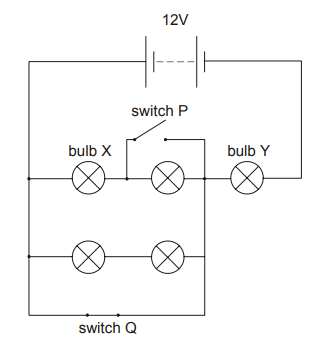Switch P is then closed and switch Q is opened.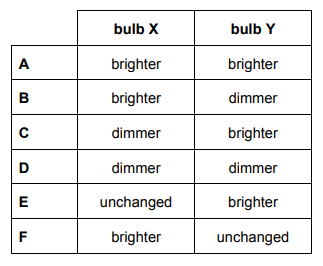15. Compared with their brightness before these changes were made, how has the brightness of bulbs X and Y changed?
0
0

## Explanation

Post Comment

A shape is formed by drawing a triangle ABC inside the triangle ADE.

BC is parallel to DE.

AB = 4cm     BC = xcm     DE = x + 3cm     DB = x – 4cm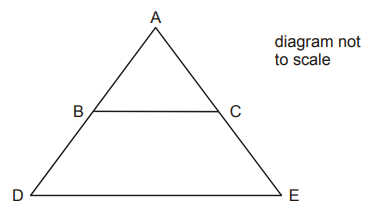16. Calculate the length of DE.
0
0

## Explanation

This question involves ratios, similar triangles and algebra. As BC and DE are parallel, similar triangles and isosceles triangles can be assumed. Set up ratios between the smaller triangle ABC and the larger triangle ADE. ABC

AB:BC 4 : x

As the multiplying factor between these ratios must be equal, find out the multiplying

factors and equalise them:

(multiply by x/4)              4 : x            (multiply by x+3/x)

x : x+3

Hence, x/4 = x+3/x

Rearrange and simplify: x2= 4(x+3) x2– 4x-12 = 0

Solve for x: (x-6)(x+2) where x=6, x= -2. As a length can never be negative, x=6

To find x+3, substitute: 6 + 3 = 9

Post Comment

In the family tree shown below, both P and Q are carriers of a recessive allele which causes a condition. Only individuals R and X have the condition.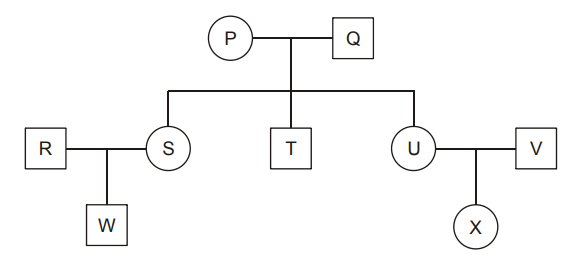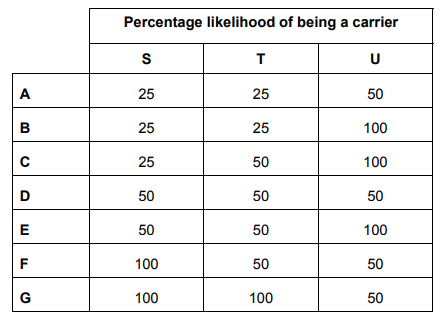17. What is the percentage likelihood of S, T and U each being a carrier?
0
0

## Explanation

This question can be worked through methodically. Let dominant allele = G, recessive allele = g.

P and Q are carriers of a recessive allele. Hence, they both have the alleles: Gg and Gg

Their offspring are:

 G g G G G Gg g Gg gg

The ratios are: 1 (GG): 2 (Gg): 1 (gg)

To deduce which of S, T, U are more likely to be carriers (Gg) we must use the further information: Only individuals R and X have the condition.

For X to have the condition, alleles of X: gg U and V cannot either be carriers (Gg) or homozygous dominant (GG), as this means that X is either a carrier or completely unaffected:

 G g G G G Gg g G G Gg

Hence, both U and V must be carriers. As the question asks for U – the percentage likelihood is 100%. The ratio of offspring is 1:2:1, with 2 (Gg). We have already found 1 Gg (U).Individual R is affected, hence its alleles are: gg For W to be unaffected, it needs to have the alleles: GG or Gg With R being gg, S needs to be either Gg or GG.

 G G g Gg Gg g Gg Gg

 G g g Gg gg g Gg gg

With S being GG, W has 100% likelihood of being a carrier, whereas with Gg, W has 50% likelihood of being a carrier. As S can either be Gg or GG, it has a 50% likelihood of being a carrier.

Now, as S and U are carriers, we have found 1 Gg (U) and 0.5 Gg (S). As the question asks for the likelihood of carriers it is plausible to assume that T also has a 50% chance of being a carrier, fulfilling the 1:2:1 ratio – 1 Gg (U), 0.5 Gg (S), ).5 Gg (T).

Post Comment

Magnesium hydrogen phosphate contains the following ions: Mg²⁺, H⁺ and PO₄ ³⁻.

18. Which one of the following is a possible formula for magnesium hydrogen phosphate?
0
0

## Explanation

For this question, the hydrogen and phosphates need to be considered first. For a neutral compound, Mg²⁺ needs to balance with a -2 charge. H⁺ and PO₄3- : 2H⁺ and PO₄³⁻ would give an overall of -1 charge (as (+2) + (3-) = -1), hence H₂PO₄. To get an a -2 charge, we need 2H₂PO₄.

Hence, (H₂PO₄)2. This -2 charge balances out with Mg²⁺, giving a neutral compound.

Hence, Mg(H₂PO₄)2.

Post Comment

The diagrams below show velocity-time or distance-time graphs for 4 different objects, P, Q, R and S.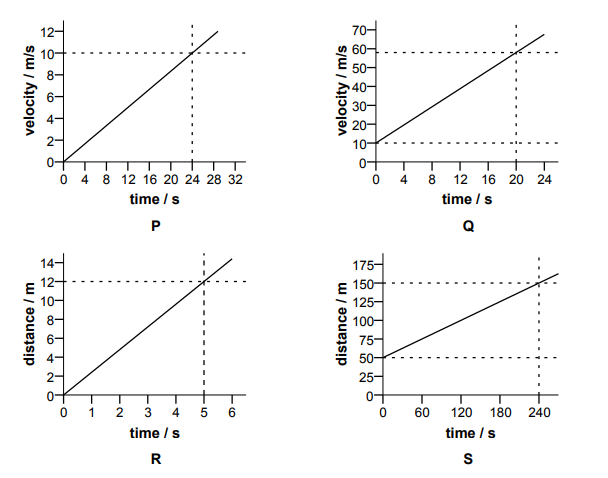19. Which graph(s) show an object accelerating at 2.4m/s²?
0
0

## Explanation

Acceleration = velocity/time = gradient of the graph

Hence, for Q: (58-10)/(20-0) = 2.4

Post CommentB Medicmind Tutor

Sun, 26 Sep 2021 14:37:51

p = 12/5 = 2.4 therefore P would also be correctB Medicmind Tutor

Sun, 26 Sep 2021 14:38:17

sorry not P i meant Rimmy Medicmind Tutor

Wed, 20 Oct 2021 15:54:33

no R is incorrect because it is a distance-time graph there the gradient=velocity= 2.4 ms-1

The total surface area of a cylinder is numerically the same as its volume.

The radius of the cylinder is r cm, the height is h cm.

20. Express h in terms of r.
0
0

## Explanation

Total Surface Area = Volume

Total Surface area = 2π

r² + 2πrh(2πas there are 2 circles; 2πrh as it’s the diameter (2r) multiplied by the height (h) and pi for the curved surface area.

Volume = πr²h

Hence, 2πr²+ 2πrh = πr²h(cancelling pi and rearranging)2r²=r²h -2rh

Factorise r and h on RHS: 2r²= rh(r-2)

Divide by r(r-2) on both sides, and obtain: 2r/(r-2)

Post Comment

The following statements are about nuclear division by meiosis and mitosis.

1 In animals meiosis only occurs in the reproductive organs.

2 Mitosis can result in the formation of clones.

3 Meiosis results in two nuclei.

4 Mitosis results in four nuclei.

5 Meiosis does not occur during asexual reproduction.

21. Which of these statements are true?
0
0

## Explanation

Statements 1,2 and 3 are fully correct – they are facts to be learned for cell division – Meiosis only occurs in reproductive organs, as the reproductive cells are fertilised to create a full set of chromosomes for the new embryo. Mitosis can result in the formation of clones, as clones are genetically identical. Asexual reproduction occurs through mitosis, not meiosis, as genetically identical organisms are produced. Statements 3 and 4 are incorrect; they should be swapped around to correct the statements: Mitosis results in 2 nuclei; Meiosis results in 4 nuclei.

Post Comment

A student prepared nitrobenzene by the following reaction.

C₆H₆ + HNO₃ → C₆H₅NO₂ + H₂O
benzene                nitrobenzene

Starting with 3.9g of benzene, the student obtained 3.69g of nitrobenzene.

(Ar : H = 1; C = 12; N = 14; O = 16)

22. What is the percentage yield?
0
0

## Explanation

To calculate the theoretical yield, as percentage yield = actual/theoretical x 100, it must be noted that the mole ratio of benzene : nitrobenzene is 1:1. Hence, calculate the moles of benzene:

n(benzene) = m/Mr = 3.9/ (6(12)+6(1)) = 0.05

0.05 is in the same moles as nitrobenzene. Hence, molar mass of nitrobenzene:

Mr (nitrobenzene): 12(6) + 5 + 14 + 16(2) = 123

Mass (nitrobenzene): 0.05 x 123 = 6.15

Hence, 3.69/6.15 x 100 = 60%

Post Comment

In an ornamental fountain, water is squirted vertically upwards through a nozzle by a pump. 5kg of water pass through the nozzle each second, and the water reaches a height of 5m after leaving the nozzle.

(Take g to be 10N/kg)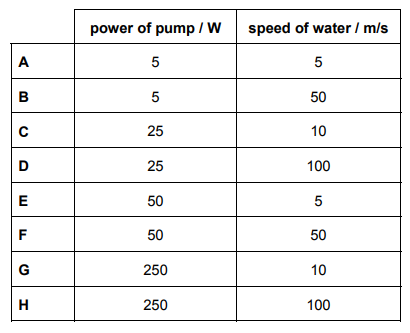23. What is the power of the pump (assuming 100% efficiency), and at what speed does the water leave the nozzle?
0
0

## Explanation

P = E/t P = mgh/t = 5 x 5 x 10 = 250 (time already included, as passing each second)

Loss of GPE = gain in Ek

Hence, 1⁄2mv2 = 250 ; v = √(2(250)/5) = 10

Post Comment

A design is set up by joining the points which are one third of the way along the sides of a square. This forms a second square as shown.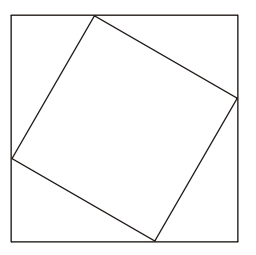This process is repeated.

24. Calculate the area of the fourth square as a fraction of the original square.
0
0

## Explanation

This question uses repeated Pythagorean theorem. It can quickly be solved by using a geometric progression (this solution will be also given, however, it will be perhaps more appreciated by A Level Mathematics students. Geometric Progression, Arithmetic Progression, or any knowledge of series beyond GCSE Mathematics is not required by the BMAT specification)

The side of the second square, forms a hypotenuse of a triangle bounded by its vertexes, and the sides of the larger square. The longer side, until the vertex of the smaller square is 2/3 of the way down. Whereas, the shorter side, until the vertex of the bigger square is 1/3 of the way down (approaching the diagram on the left), and opposite for the right hand side.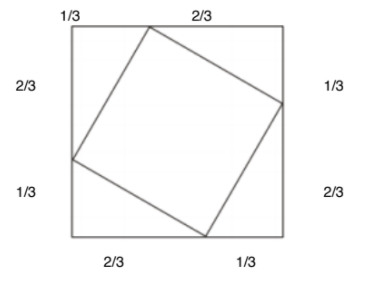The hypotenuse can be calculated via Pythagoras: √( (2/3)²+(1/3)²) = √5/3

As the process is repeated, the 2nd square is in the same arrangement as above, however, the lengths expressed are: 1/3x(√5/3) and 2/3x(√5/3).

Hence, the length of the side of the 3rd square:

(1/3x(√5/3))²+(2/3x(√5/3))²) = √(5/81 + 20/81) = √(25/81)

As the process is repeated, the 3rd square is in the same arrangement as above, however, the lengths expressed are: 1/3x(√(25/81)) and 2/3x(√(25/81)).

Hence, the length of the side of the 4th square:

√((1/3x√(25/81))²+(2/3x√(25/81))²) = √(25/729 + 100/729) = √(125/729)

The length of the side of the 4th square can be squared, to give the area.

Hence, (√(125/729))²= 125/729

(Geometric Progression

Let the side of the first square = a, hence its area = a². The common ratio, r, between each term = 5/9, as the ratio between the side of length a and the second square = 5/9, which was deduced from Pythagoras. To find the area of the 4th square, the formula for a GP => =a², n=4, r=5/9

Hence, Area of 4th square = a² x (5/9)4-1 = a x (5/9)3 = 125/729a²

Post Comment

The hormonal and nervous systems are often compared because of their similar roles in the body.

1 The nervous system relies only on electrical impulses to work.

2 Both the hormonal and nervous systems activate target structures.

3 The fastest responses in the body are produced by the nervous system.

4 Only the hormonal system relies on the activity of chemicals.

5 Parts of the hormonal system may be controlled by the central nervous system.

25. Which of the following statements about the two systems are true?
0
0

## Explanation

Only Statements 2,3 and 5 are correct. Statement 1 and 2 are incorrect – the nervous system uses neurotransmitter chemicals at synapses, to continue an electrical impulse across another neurone.

Both hormonal and nervous systems activate specified targets i.e. the nervous system activates an effector (muscle in a reflex response), hormonal system stimulates an organ/tissue/localised area for a particular function (ADH controlling reabsorption of water in kidneys). Parts of the hormonal system may be controlled by the nervous system, as effectors can be glands (i.e. thermoreceptors on the skin detect temperature changes, and a nervous impulse is carried to the thermoregulatory centre)

Post Comment

Cyclohexene, C6H10, is drawn as: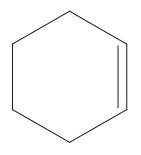Apply this information for the steroid-type structure shown below to find the total number of carbon atoms in the molecule.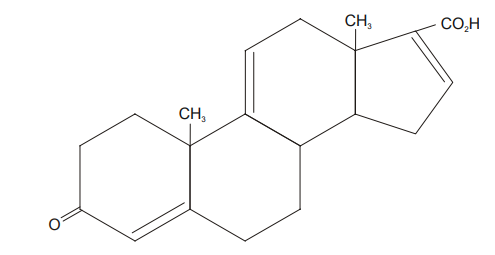26.
0
0

## Explanation

At each junction between 2 or more lines there is a carbon atom. This makes a total of 17 carbon atoms not drawn on the diagram, plus the additional 3 carbon atoms which are drawn on, making a total of 17+3=20 carbon atoms.

Post Comment

A car of mass 800kg moves up an incline of 1 in 20 (1 in 20 means for every 20m along the road the car gains 1m in height) at a constant speed of 20m/s. The frictional force opposing motion is 500N.

27. How much work has been done by the engine after the car has moved 50m?
0
0

## Explanation

Energy + Work Done against frictional force = Total Energy

According to the ratio of 1/20, the vertical height after moving 50m: 1/20 x 50 = 5/2

Hence, GPE = mgh = 800 x 10 x (5/2) = 2000J

Work done to overcome friction = 500N x 50m = 2500J

Hence, 2500 + 2000 = 4500J

Post CommentP Medicmind Tutor

Tue, 02 Nov 2021 10:10:37

Hi, in the solution it says that 800 x 10 x (5/2) = 2000J but the answer should be 20 000J, similarly, it also says 500N x 50m = 2500J whereas it should be 25 000J. This would make more sense as 4 500J would 4.5kJ not 45kJ which is the correct answer

## Instructions

Below is a summary of your answers. You can review your questions in three (3) different ways.

The buttons in the lower right-hand corner correspond to these choices:

2. Review questions that are incomplete.
3. Review questions that are flagged for review. (Click the 'flag' icon to change the flag for review status.)

You may also click on a question number to link directly to its location in the exam.

## Instructions

This review section allows you to view the answers you made and see whether they were correct or not. Each question accessed from this screen has an 'Explain Answer' button in the top left hand side. By clicking on this you will obtain an explanation as to the correct answer.

At the bottom of this screen you can choose to 'Review All' answers, 'Review Incorrect' answers or 'Review Flagged' answers. Alternatively you can go to specific questions by opening up any of the sub-tests below.

x

## When should we call you?

It would be great to have a 15m chat to discuss a personalised plan and answer any questions

## What time works best for you? (UK Time)

Pick a time-slot that works best for you ?

Submit

Submit